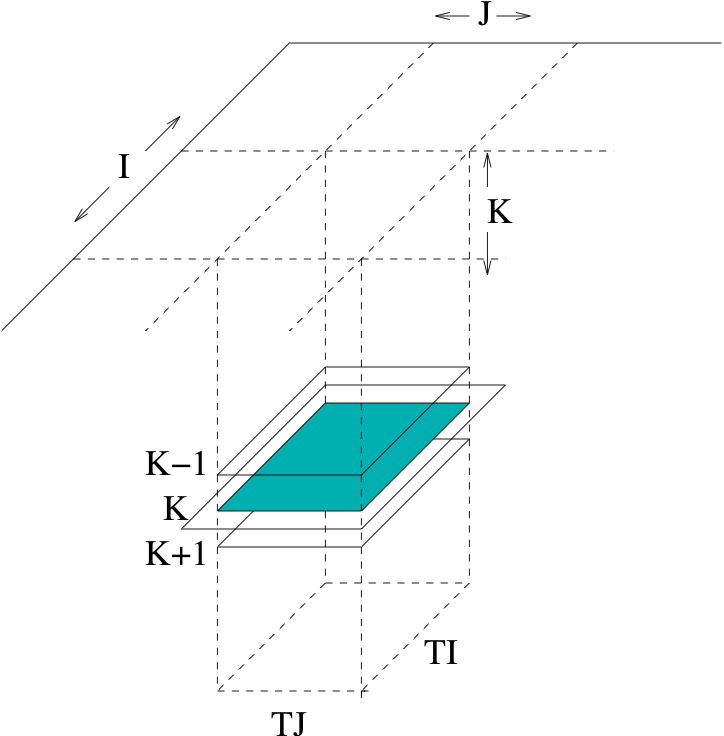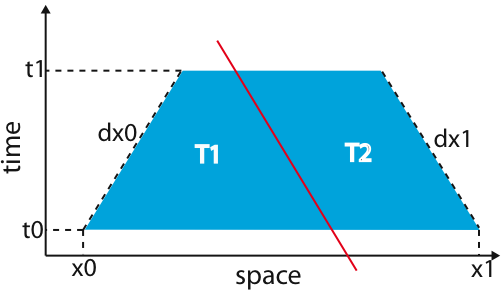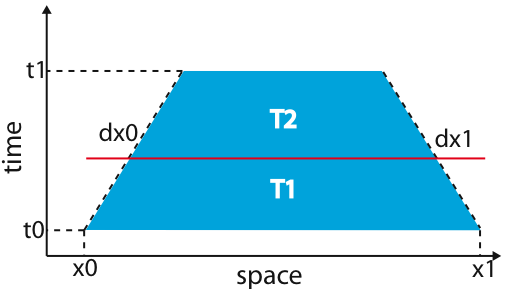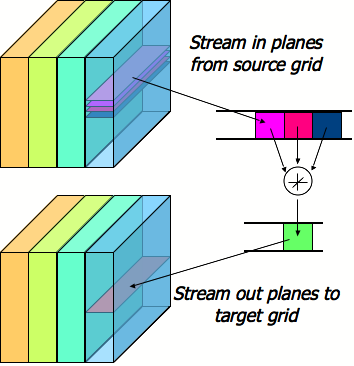# StencilProbe: A Microbenchmark for Stencil Applications

The Stencil Probe is a small, self-contained serial microbenchmark that we developed as a tool to explore the behavior of grid-based computations. As such it is suitable for experimentation on architectures in varying states of implementation -- from production CPUs to cycle-accurate simulators. By modifying the operations in the inner loop of the benchmark, the Stencil Probe can effectively mimic the kernels of applications that use stencils on regular grids. In this way, we can easily simulate the memory access patterns and performance of large applications, as well as use the Stencil Probe as a testbed for potential optimizations, without having to port or modify the entire application.

The latest development version of StencilProbe is available via a public git repository:

```      git clone http://github.com/shoaibkamil/stencilprobe.git
```
Snapshots will be available soon.

## Details

The current version of the StencilProbe contains code for four different optimization strategies, for a simple 3D 7-point stencil derived from the heat equation: 2D cache blocking, time skewing, cache oblivious, and circular queue. This section describes the various optimization techniques. Generally, for instructions/how to run, run the executable with no arguments.

### 2D Cache Blocking (due to Rivera et al)

In , Rivera and Tseng propose a 2D blocking for a 3D stencil, observing that a 3D blocking would result in very small dimensions for the block. The 2D blocking is as shown in the diagram below, where I is the unit-stride dimension--- note that the blocking is in the two most-unit-stride dimensions.Figure 1: 2D "Rivera" blocking, where I is the unit-stride dimension.

To run this version of the code, use the "blocked_probe" makefile target. This implementation supports arbitrary block sizes; that is, the block size in any dimension does not need to be a divisor of the grid size. In addition, the blocksize in the k-dimension is ignored.

### Cache Oblivious

The cache oblivious stencil algorithm  performs cache blocking implicitly, using recursion, instead of implicit cache blocking as above, and utilizes blocking in all dimensions, including time. The version of the code in the StencilProbe is from  and includes optimizations to eliminate blocking in the unit-stride dimension as well as implementing a "cutoff" parameter to stop the recursion once the block is sufficiently small.

The algorithm operates on an (n+1)-dimensional (where n is the dimension of the stencil) spacetime trapezoid, cutting it in either the space or time dimensions at each step, or performing a computation if either the current block is small or if no further cuts are possible. For example, consider the one-dimensional space example below, which is a two-dimensional spacetime trapezoid.Figure 2: 2D spacetime trapezoid (1D in space, 1D in time).

The horizontal axis is the space dimension, and the vertical axis is the time dimension, and dx0 and dx1 are dependent on the stencil footprint--- they represent the dependencies between time steps. At each step, if the the remaining space dimension is long enough, the code recurses by splitting the trapezoid and preserving time-space dependencies, as below.Figure 3: Cache oblivious space cut.

However, if the remaining trapezoid is "tall and skinny," the code takes a recursive cut in the time dimension, splitting the trapezoid as below.Figure 3: Cache oblivious time cut.

The cuts proceed until there is only one timestep remaining or if the remaining block size is sufficiently small, at which point all the computation that is possible occurs on the block. The cutoff size can be set in the file "run.h". Note that the executable for this version ignores the blocking parameters (as the blocking is recursive and implicit).

### Time Skewing

Time skewing is a type of cache tiling that attempts reduce main memory traffic by reusing values in cache as often as possible. Figure 4 shows a simplified diagram of time skewing for a 3-point stencil:Figure 4: Time skewing in 2D spacetime.

The grid is divided into cache blocks by several skewed cuts, similar to the space cuts from the cache oblivious algorithm. These cuts are skewed in order to preserve the data dependencies of the stencil. For example, the cut between the first and second cache blocks allows the first cache block to be fully calculated before starting on the second cache block. In general, this holds true between the nth and (n+1)th cache blocks. As long as the blocks are executed in the proper order, the algorithm respects the stencil dependencies.

However, the blocks generated from time skewing do not all perform the same amount of work, despite being equally partitioned initially. For instance, the points in Figure 4 are equally divided for the first time step. However, as time progresses, the shifting causes the cache blocks at the boundaries to perform unequal work. The number of points per iteration slowly decreases for the first cache block, while it slowly increases for the final cache block. For interior cache blocks, the shifting does not change the number of points per iteration, and so they all perform the same number of stencil operations.

There are two major points of concern caused by this shifting. The first is that extra cache misses may be incurred, thereby hindering our efforts to minimize memory traffic. Fortunately, this shift is always towards the completed portion of the grid, so the needed points are often already resident in cache. This helps in mitigating, if not eliminating, the extra memory traffic.

The second concern is that the shifting limits the number of iterations that can be performed. Specifically, some of the cache blocks along the boundary can be shifted off the grid as time progresses. Once a cache block is off the grid, any further iterations will cause dependency violations. This is seen in Figure 4, where the first cache block shifts completely over the boundary after the third iteration. In these cases, we can perform a time cut (as explained in Figure 3) to ``restart" the algorithm. After the time cut, we can either execute the remaining number of iterations or, if needed, perform another time cut. Of course, this problem can also be addressed by simply using a larger cache block.

A closer representation to our actual 3D time skewing code is illustrated below:Figure 4: Time skewing in 4D spacetime, where four iterations are performed. Each cache block is shown separately.

By showing how the number of stencil operations performed varies within each cache block, the diagram sheds light on how time skewing works in higher dimensions.

### Circular Queue

The circular queue algorithm employs a separate "circular queue" data structure to perform the actual stencil calculations:Figure 6: One iteration of the circular queue algorithm, where each colored slab represents a separate cache block.

The circular queue data structure stores only as many planes as is needed for the given stencil. In the case of the 3D 7-point stencil, 3 read planes are required to compute one write plane (as shown in Figure 6). As a result, the circular queue data structure only stores 3 read planes. After completing a plane, the pointer to the lowest read plane is moved to point to the new top read plane, thus making it a "circular" queue.

In general, the circular queue stores (t-1) sets of planes, where t is the number of iterations performed. Thus, a separate data structure is not needed if only a single iteration is being performed.

There are several advantages to the circular queue algorithm. First, the algorithm is easily parallelizable. Unlike time skewing, each of the cache blocks can be assigned to a different processor without worrying about dependencies. Second, the algorithm exploits reuse across several iterations. The circular queue data structure is designed this way, assuming the cache block size is chosen appropriately. Finally, there is no need to alternate the source and target grids after each iteration. The circular queue takes care of this, and only the final result is written to the target grid.

There are however, a few drawbacks with the algorithm. First, there will be redundant computation if more than one iteration is being performed. This is exacerbated if the cache blocks are small or if many iterations are being performed. Second, like time skewing, we still need to search for the optimal cache block size. This might be done via a heuristic, performance model, or exhaustive search. Finally, a separate data structure needs to be allocated. While the memory footprint for the circular queue is typically small, it is proportional to the cache block size.

## References

 G. Rivera and C. Tseng. Tiling optimizations for 3d scientific computations. In Proceedings of SC'00, Dallas, TX, November 2000. Supercomputing 2000.
 M. Frigo and V. Strumpen. Cache oblivious stencil computations. Proceedings of the 19th annual international conference on Supercomputing.
 D. Wonnacott. Using time skewing to eliminate idle time due to memory bandwidth and network limitations. In IPDPS:Interational Conference on Paral lel and Distributed Com- puting Systems, Cancun, Mexico, 2000.